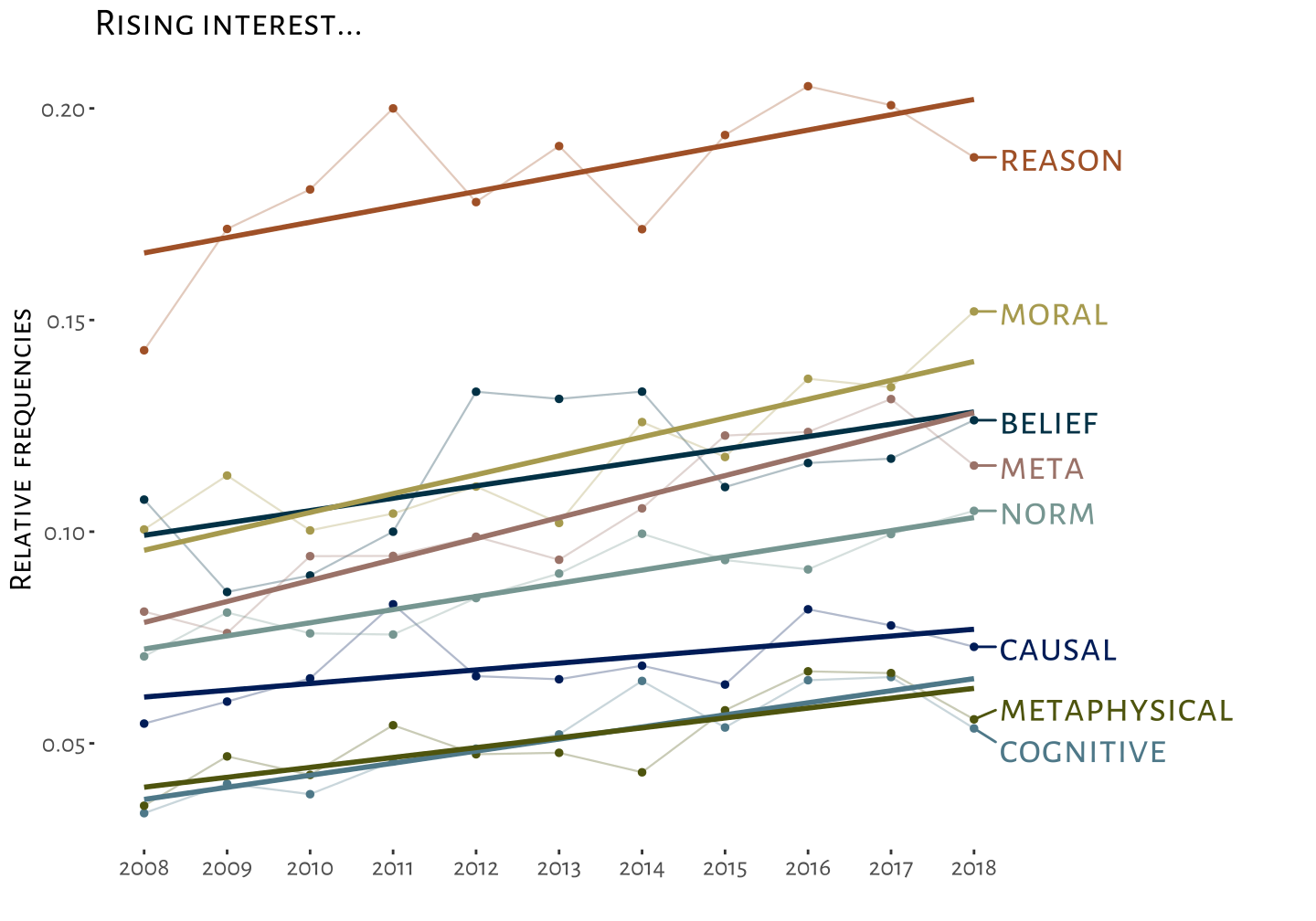``````#install.packages("Cairo")
knitr::opts_chunk\$set(dev="CairoPNG", fig.width=10, fig.height=7, dpi = 72)
options(digits = 4)``````

Recently I read this very good exploration by Eric Schwitzgebel.He was looking through the philosophers index to track the lives of certain philosophical terms – when they where hot, and when they began to cool down. I thought that was pretty neat, but it left me wondering: Would it not be interesting to look at a more recent period, let’s say, ten years, and find out which terms increased the most in use, and which are, right now, loosing popularity. So that’s what I did: I collected around 8000 abstracts from the Web of Science, the complete records for the last ten years of the top 30 Journals from this list.. I tokenized them into (n=1,2,3)-grams, kept the top thousand of each n, calculated for each the linear regression of (normalized) occurences over time, and ordered them by the resulting slope. The Python code is on the bottom of the page.

Let us have look! The single words with the strongest increase are:

``````#install.packages("rlang")
library(ggplot2)
library(tidyr)
#devtools::install_github("hrbrmstr/hrbrthemes")
#library(hrbrthemes)
#install.packages("tidyverse")
#library(gcookbook)
#devtools::install_github("slowkow/ggrepel")

library(ggrepel)
#library(tidyverse)

require(showtext)
showtext.auto()

library(dplyr)
theme_set(theme_classic())

myPal <- c("#023145",
"#001c57",
"#4e7888",
"#9a7268",
"#4e540e",
"#a69a4e",
"#769691",
"#9f5027",
"#f8c760",
"#3c4154",
"#92aacc",
"#596e9b")

myfall <- c(
"#571012",
"#545f52",
"#48320d",
"#9f0011",
"#051626",
"#b07e1f",
"#6b2a0a",
"#2e5465",
"#020100"
)
newfall <- c("#001c57",
"#769691",
"#9f5027",
"#f8c760",
"#92aacc",
"#596e9b")
#df <- df[sample(nrow(df), 3), ]
c <- c("meta","moral","reason","belief","norm","metaphysical","cognitive","causal")
#c<- c("cognitive science","natural kinds","causal explanation","mechanistic explanation","perceptual experience","virtue epistemology","epistemic value","decision theory","moral realism")

#c <- c("scientific realism","natural selection","personal identity","structural realism","modal logic","epistemic logic")
#c <- c("moral error theory","life worth living","high energy physics","second order logic","normative decision theory","scientia mensura principle","causal decision theory","greenhouse gas emissions","homeostatic property cluster")
#c <- c("quantum field theory","dynamic epistemic logic","natural language semantics","ontic structural realism")

names(df) <- sub("X", "", names(df))
df <- df[df\$word %in% c,]
df <- gather(df, key=year, value,-word)

p <- ggplot(data = df, mapping = aes(x = year, y = value, group=word))

p + geom_point(aes(color=word), size=1.2) +
geom_line(aes(color=word),size=0.4, alpha=0.3) +
scale_color_manual(values = myPal) +
geom_smooth(method='lm',aes(color=word), se=FALSE)+
labs(title="Rising interest...", family = "Alegreya Sans SC", size = 45)+
xlab("") + ylab("Relative  frequencies") + theme(legend.position="none")+

geom_text_repel(data = df[df\$year == "2018", ], aes(label = word, color=word), direction="y",hjust=0, nudge_x=0.3,family = "Alegreya Sans SC", size = 16)+

theme(text = element_text(family = "Alegreya Sans SC", size = 35))+
expand_limits(x = 15)+
theme(axis.line = element_line(colour="white"))``````I curated the above list a bit to exclude meaningless words. It seems like philosophers have a pretty strong newfound interest in things that “metaphysical”, and increasingly believe that “moral” is an important thing. – More telling than the biggest increases are in this case, I think, the words with the biggest decrease: The words that philosophers were very interested in ten years ago, and in which they then lost interest rapidly:

``````library(grid)

names(df) <- sub("X", "", names(df))

df <- df[df\$word %in% c,]
last_vals <- df\$"2018"
words <- c(df )

df <- gather(df, key=year, value,-word)

p <- ggplot(data = df, mapping = aes(x = year, y = value, group=word))

p + geom_point(aes(color=word), size=1.2) +
geom_line(aes(color=word),size=0.4, alpha=0.3) +
scale_color_manual(values = myfall) +
geom_smooth(method='lm',aes(color=word), se=FALSE)+
labs(title="Falling interest...", family = "Alegreya Sans SC", size = 45)+
xlab("") + ylab("Relative  frequencies") + theme(legend.position="none")+

geom_text_repel(data = df[df\$year == "2018", ], aes(label = word, color=word), direction="y",hjust=0, nudge_x=0.3,family = "Alegreya Sans SC", size = 16)+

theme(text = element_text(family = "Alegreya Sans SC", size = 35))+
expand_limits(x = 15)+
theme(axis.line = element_line(colour="white"))``````